# Solv manifold

(diff) ← Older revision | Latest revision (diff) | Newer revision → (diff)

solvmanifold, solvable manifold

A homogeneous spaceof a connected solvable Lie group(cf. Lie group, solvable). It can be identified with the coset space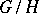, whereis the stabilizer subgroup of some point of the manifold.

Examples:, the torus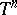, the Iwasawa manifold(whereis the group of all upper-triangular matrices with 1's on the main diagonal in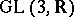andis the subgroup of all integer points in),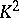(the Klein bottle), and(the Möbius band).

The first solvmanifolds studied were those in the narrower class of nil manifolds (cf. Nil manifold), that is, homogeneous spaces of nilpotent Lie groups (such as,,, but notand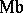). The following results are due to A.I. Mal'tsev (see ). 1) Every nil manifold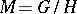is diffeomorphic to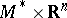, whereis a compact nil manifold. 2) Ifis compact andacts effectively on, then the stabilizeris a discrete subgroup. 3) A nilpotent Lie group(cf. Lie group, nilpotent) acts transitively and locally effectively on some compact manifold if and only if its Lie algebrahas a-form. If, in addition,is simply connected, then it is isomorphic to a unipotent algebraic group defined overandis an arithmetic subgroup of. 4) The fundamental group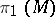of a compact nil manifold(which is isomorphic towhenis simply connected and its action onis locally effective) determines it up to a diffeomorphism. The groupsthat can arise here are just the finitely-generated nilpotent torsion-free groups.

These results admit partial generalizations to arbitrary solvmanifolds. Thus, for any solvmanifoldthere is a solvmanifold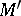which is a finitely-sheeted covering of it and is diffeomorphic to, whereis some compact solvmanifold. An arbitrary solvmanifold cannot always be decomposed into a direct product, but it is diffeomorphic (see , ) to the space of a vector bundle over some compact solvmanifold (forthe corresponding bundle is a non-trivial line bundle over). The fundamental groupof an arbitrary solvmanifoldis polycyclic (cf. Polycyclic group), and ifis compact, it determinesuniquely up to a diffeomorphism. A groupis isomorphic tofor some compact solvmanifoldif and only if it is contained in an exact sequence of the formwhereis a finitely-generated nilpotent torsion-free group. Every polycyclic group has a subgroup of finite index that is isomorphic tofor some compact solvmanifold. If a solvable Lie groupacts transitively and locally effectively on a compact solvmanifold, thenis fibred over a torus with fibre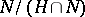, whereis the nil radical of. A solvmanifoldis compact if and only if there is a-invariant measure onwith respect to which the volume ofis finite.

Every solvmanifoldis aspherical (that is, the homotopy groupfor). Among all compact homogeneous spaces, compact solvmanifolds are characterized by asphericity and the solvability of(see ).

How to Cite This Entry:
Solv manifold. Encyclopedia of Mathematics. URL: http://encyclopediaofmath.org/index.php?title=Solv_manifold&oldid=14146
This article was adapted from an original article by V.V. Gorbatsevich (originator), which appeared in Encyclopedia of Mathematics - ISBN 1402006098. See original article